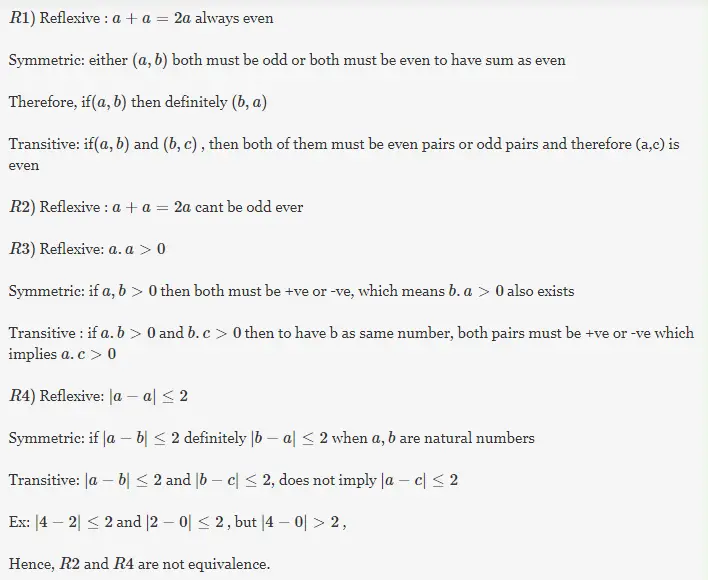# Gate CS-2001 Question Paper With Solutions

Q. 2 Consider the following relations:

```R1(a,b) iff (a+b) is even over the set of integers
R2(a,b) iff (a+b) is odd over the set of integers
R3(a,b) iff a.b > 0 over the set of non-zero rational numbers
R4(a,b) iff |a - b| <= 2 over the set of natural numbers```

Which of the following statements is correct?

(A) R1 and R2 are equivalence relations, R3 and R4 are not

(B) R1 and R3 are equivalence relations, R2 and R4 are not

(C) R1 and R4 are equivalence relations, R2 and R3 are not

(D) R1, R2, R3 and R4 are all equivalence relations